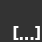# …Details…Thoughts that's escape, only details.

## OpenVMS – SCSSYSTEMID

SCSSYSTEMID (G)
Specifies a number that identifies the computer. This parameter is not dynamic. SCSSYSTEMID is the low-order 32 bits of the 48-bit system identification number.

If the computer is in an OpenVMS Cluster, specify a value that is unique within the cluster. Do not use zero as the value.

If the computer is running DECnet for OpenVMS, calculate the value from the DECnet address using the following formula:

SCSSYSTEMID = ((DECnet area number) * 1024) + (DECnet node number)

Example: If the DECnet address is 2.211, calculate the value as follows:

SCSSYSTEMID = (2 * 1024) + 211 = 2259

Category: VMS

Tagged: ,